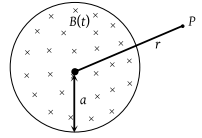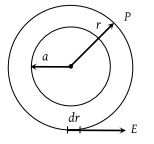NEET Questions Solved

A uniform but time-varying magnetic field B(t) exists in a circular region of radius a and is directed into the plane of the paper, as shown. The magnitude of the induced electric field at point P at a distance r from the centre of the circular region(1) Is zero

(2) Decreases as $\frac{1}{r}$

(3) Increases as r

(4) Decreases as $\frac{1}{{r}^{2}}$

(2) Construct a concentric circle of radius r. The induced electric field (E) at any point on the circle is equal to that at P. For this circle$\oint \stackrel{\to }{E}.d\stackrel{\to }{l}=\left|\frac{d\varphi }{dt}\right|=A\text{\hspace{0.17em}}\left|\frac{dB}{dt}\right|$

or $E×\left(2\pi r\right)=\pi {a}^{2}.\left|\frac{dB}{dt}\right|$

$⇒E=\frac{{a}^{2}}{2r}\left|\frac{dB}{dt}\right|⇒E\propto \frac{1}{r}$

Difficulty Level:

• 13%
• 63%
• 10%
• 15%